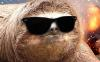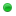Everyone who has never used our Bots before, can test each one for 2 days without any limitation.
The trial is given automatically when you login on the Bot, but in some cases it wouldn't work (security reasons).
If this happens, send me a private message and i will be checking the failed trials manually and adding it for those who didn't get it.
We are looking for resellers who may accept payment methods different from ours, including classictibia's cash, realesta's cash, mastercores' cash, etc. Interested? Click here at anytime.

 FREE Follow multiple floorsArkilysAdministratorPosts: 2,619 Threads: 429 Joined: Jul 2018 Reputation: 69 09-17-2021, 04:28 PM Follow multiple floors SETUP 1. Player = Player name that you will follow. 2. Monsters = Monsters list that bot should consider to follow/unfollow player. 3. LADDERS_IDS, UP_STAIRS_IDS, DOWN_STAIRS_IDS = Ids of ladders, stairs that goes up stairs and stairs/holes that goes downstairs. The script already tries to guess and find ladders, stairs, etc. based on some item flags, but I'm not sure if it will be 100% accurate. So I've added those lists to let people manually add those items if the script is not identifying correctly. Warnings I just tested it worked on simple tests like going upstairs or not, I didn't tested on real hunting or so. So be careful while hunting with this script, I strongly recommend you to watch it before going AFK. I recommend you to use this script with low intervals on Persistent, so it may work faster. If you are going to bot two characters, make sure the "leader" will wait for the secondary (characters that follows leader). I'm not sure if this script will work fine when going to the city or so. (COPY)```local Player = 'Player name' local Monsters = { 'Demon', 'Demon Skeleton', 'Ghoul' } local LADDERS_IDS = { 1948, } -- Ladder ids. The ones that you must USE to go upstairs. local UP_STAIRS_IDS  = { 1947 } -- Stairs ids that goes upstairs. local DOWN_STAIRS_IDS = { 411, 413 } -- Stairs and holes ids that goes downstairs. local monsters_around = maroundreachable(0, table.unpack(Monsters)) if monsters_around == 0 and target().id == 0 then     local follow_creature = followed()     if follow_creature.id == 0 or (follow_creature.id > 0 and follow_creature.name:lower() ~= Player:lower()) then         if paround(0, Player) > 0 then             follow(Player)         else             local creatures = getcreatures('p')             local FLOOR_DIFF = -1             for _, creature in ipairs(creatures) do                 if creature.name:lower() == Player:lower() then                     FLOOR_DIFF = posz() - creature.posz                     break                 end             end             if FLOOR_DIFF ~= 0 then                 local player_x = posx()                 local player_y = posy()                 local player_z = posz()                 local closest_dist = -1                 local sqm_reach = nil                 local tiles = gettiles()                 for _, tile in ipairs(tiles) do                     if tile.posz == player_z then                         for __, item in ipairs(tile.items) do                             if item.id >= 100 then                                 if (FLOOR_DIFF < 0 or FLOOR_DIFF == -1) and table.find(DOWN_STAIRS_IDS, item.id) ~= nil then                                     if tilereachable(tile.posx, tile.posy, tile.posz) then                                         if FLOOR_DIFF == -1 then                                             local dist = proximity(player_x, player_y, tile.posx, tile.posy)                                             if dist < closest_dist or closest_dist == -1 then                                                 closest_dist = dist                                                 sqm_reach = { posx = tile.posx, posy = tile.posy, posz = tile.posz }                                             end                                         else                                                     sqm_reach = { posx = tile.posx, posy = tile.posy, posz = tile.posz }                                         end                                         break                                     end                                 elseif (FLOOR_DIFF > 0 or FLOOR_DIFF == -1) and table.find(LADDERS_IDS, item.id) ~= nil then                                     if tilereachable(tile.posx, tile.posy, tile.posz) then                                         if FLOOR_DIFF == -1 then                                             local dist = proximity(player_x, player_y, tile.posx, tile.posy)                                             if dist < closest_dist or closest_dist == -1 then                                                 closest_dist = dist                                                 sqm_reach = { posx = tile.posx, posy = tile.posy, posz = tile.posz, ladder = item.id }                                             end                                         else                                                     sqm_reach = { posx = tile.posx, posy = tile.posy, posz = tile.posz, ladder = item.id }                                         end                                         break                                     end                                 elseif (FLOOR_DIFF > 0 or FLOOR_DIFF == -1) and table.find(UP_STAIRS_IDS, item.id) ~= nil then                                     if tilereachable(tile.posx, tile.posy, tile.posz) then                                         if FLOOR_DIFF == -1 then                                             local dist = proximity(player_x, player_y, tile.posx, tile.posy)                                             if dist < closest_dist or closest_dist == -1 then                                                 closest_dist = dist                                                 sqm_reach = { posx = tile.posx, posy = tile.posy, posz = tile.posz }                                             end                                         else                                                     sqm_reach = { posx = tile.posx, posy = tile.posy, posz = tile.posz }                                         end                                         break                                     end                                 else                                     local flags = itemflags(item.id)                                     if itemhasflags(item.id, 0, 13, 15, 23) and (FLOOR_DIFF < 0 or FLOOR_DIFF == -1) then                                         if tilereachable(tile.posx, tile.posy, tile.posz) then                                             if FLOOR_DIFF == -1 then                                                 local dist = proximity(player_x, player_y, tile.posx, tile.posy)                                                 if dist < closest_dist or closest_dist == -1 then                                                     closest_dist = dist                                                     sqm_reach = { posx = tile.posx, posy = tile.posy, posz = tile.posz }                                                 end                                             else                                                         sqm_reach = { posx = tile.posx, posy = tile.posy, posz = tile.posz }                                             end                                         end                                     elseif itemhasflags(item.id, 6) and (FLOOR_DIFF > 0 or FLOOR_DIFF == -1) then                                         if tilereachable(tile.posx, tile.posy, tile.posz) then                                             if FLOOR_DIFF == -1 then                                                 local dist = proximity(player_x, player_y, tile.posx, tile.posy)                                                 if dist < closest_dist or closest_dist == -1 then                                                     closest_dist = dist                                                     sqm_reach = { posx = tile.posx, posy = tile.posy, posz = tile.posz }                                                 end                                             else                                                         sqm_reach = { posx = tile.posx, posy = tile.posy, posz = tile.posz }                                             end                                         end                                     elseif itemhasflags(item.id, 13, 15, 25, 28, 29) and (FLOOR_DIFF > 0 or FLOOR_DIFF == -1) then                                         if tilereachable(tile.posx, tile.posy, tile.posz) then                                             if FLOOR_DIFF == -1 then                                                 local dist = proximity(player_x, player_y, tile.posx, tile.posy)                                                 if dist < closest_dist or closest_dist == -1 then                                                     closest_dist = dist                                                     sqm_reach = { posx = tile.posx, posy = tile.posy, posz = tile.posz, ladder = item.id }                                                 end                                             else                                                         sqm_reach = { posx = tile.posx, posy = tile.posy, posz = tile.posz, ladder = item.id }                                             end                                         end                                     end                                 end                             end                         end                     end                 end                 if sqm_reach ~= nil then                     reachlocation(sqm_reach.posx, sqm_reach.posy, sqm_reach.posz, true)                     wait(200, 400)                     if sqm_reach.ladder ~= nil then                         useitem(sqm_reach.ladder, ground(sqm_reach.posx, sqm_reach.posy, sqm_reach.posz))                         wait(800, 1200)                     end                     if paround(0, Player) > 0 then                         follow(Player)                     end                 end             end         end     end elseif monsters_around > 0 and followed().id > 0 then     stop() end``` keywords: follow player, follow friend, follow bot, follow field, follow different floor, follow multifloor, follow flor